# Opinion of Prof. Vadim Utkin

### 4. Finite time convergence

4.1.HOSM was announced as an efficient tool for chattering suppression, for example chattering caused by unmodeled dynamics. But at the presence of chattering finite time convergence is not of any value. Convergence to what?

4.2. HOSM implies finite time convergence to manifold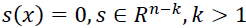, and then sliding mode in this manifold. But if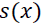is Lipschitzian function, then convergence in sliding mode is asymptotic (not finite time) and the total process is converging asymptotically. Then finite time of the first stage (reaching sliding manifold) is not of any value: the processes in HOSM systems are not converging in finite time, but asymptotically only. Then the processes with the same rate of convergence can be easily implemented by “old” sliding mode control. But “old” control is much simpler than HOSM control. Please compare two control functions of the same arguments resulting in the same processes for the case k=3

“Old” control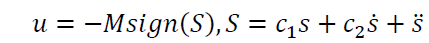HOSM control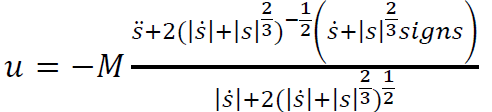The case n=3, k=2 is shown in Fig. 5 with green (“old” control) and red (HOSM control)
trajectories.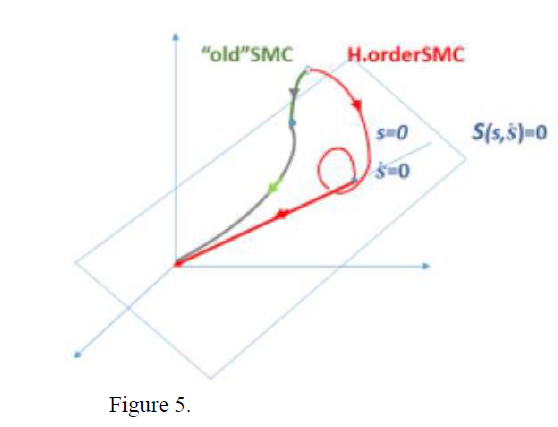### 5. Finite time convergence and chattering.

As stated in the previous section “old” and HOSM controls have similar processes, but implementation of the first one is much simpler. These two approaches cannot be compared when function